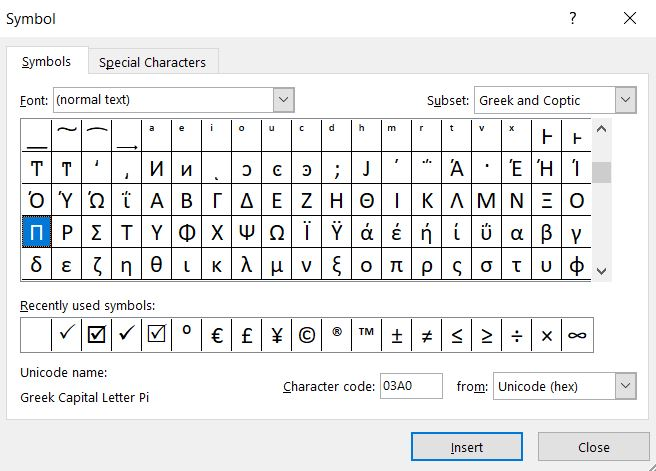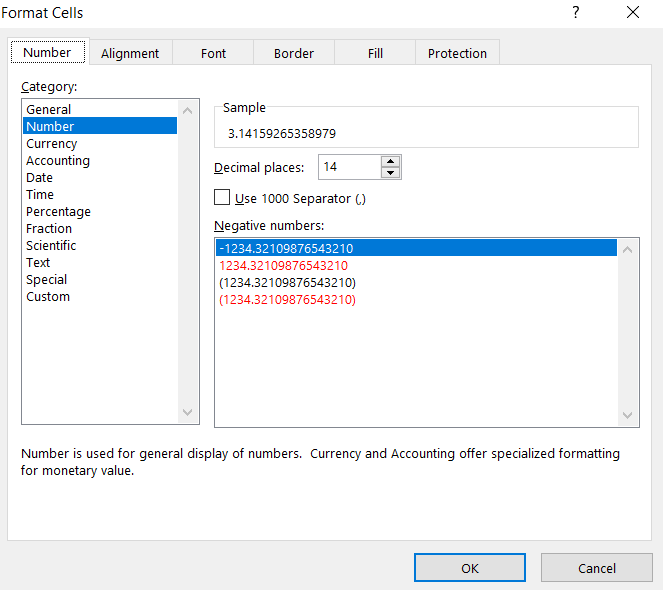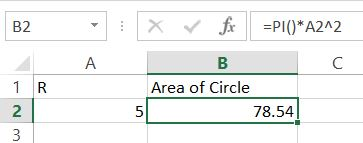#### How to Use Pi in Excel

In this Excel article, you learn how to use Pi in Excel.

The π is a special value known since ancient times. The pi value represents a half-rotation in the radian angle system.

In Excel you can use pi value for geometric calculations.

## Pi function in Excel

Let’s start from using pi in Excel. Syntax of PI function is very easy. There are no arguments of pi function.

Excel PI formula is just =PI()

Excel Pi function returns pi value up to 15 digits.

π in Excel equals exactly 3.14159265358979 which is the exact value of this constant.

## How to type pi in Excel?

You can insert π symbol in Excel. To do that go to Insert tab and click Symbol button.Greek capital letter Pi you can find in Greek and Coptic subset.

## How to display an exact value of Pi?

To show every fifteen digits of the Pi right-click a cell and select Format Cells option.

`You can also use a keyboard shortcut of Alt + 1.`

From the Format Cells menu pick Number and increase decimal places to 14. The Pi equals to fifteen digits which is three plus fourteen digits (exactly 3.14159265358979).In the same cell type =PI() and press Enter keyboard button.

Probably, instead of Pi value you will see just ### in the cell. This is because your cell to narrow. You need to increase the size of the cell. Just drag the header of the spreadsheet drag to enlarge the column. Now, you can see the every fifteen digits of Pi.

## The Pi in VBA

Calculating the value of Pi (π) in Excel using VBA (Visual Basic for Applications) involves approximating it through mathematical formulas. One common method is to use the Leibniz formula for Pi, which converges to Pi as you calculate more terms.

```Function CalculatePi(Iterations As Integer) As Double
Dim i As Integer
Dim PiApproximation As Double
PiApproximation = 0
For i = 0 To Iterations
PiApproximation = PiApproximation + ((-1) ^ i) / (2 * i + 1)
Next i
CalculatePi = PiApproximation * 4
End Function
```

This code defines a custom Excel function called CalculatePi. It uses the Leibniz formula to approximate Pi based on the number of iterations specified.

Now, you can use the CalculatePi function in your Excel spreadsheet. In any cell, type a formula like this:

`=CalculatePi(1000)`

This formula will calculate an approximation of Pi using 1000 iterations. You can change the number of iterations to increase the accuracy of the approximation. However, keep in mind that extremely high iteration counts can be computationally intensive.

## Example of using pi in Excel

You can use pi function to calculate area of the circle. Use this pi Excel formula

=PI()*A2^2